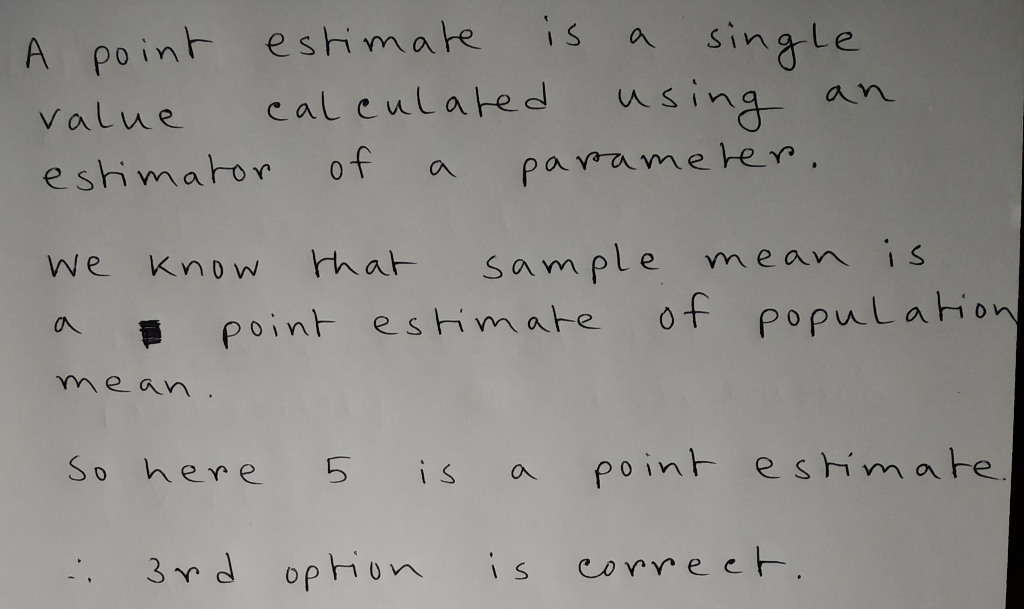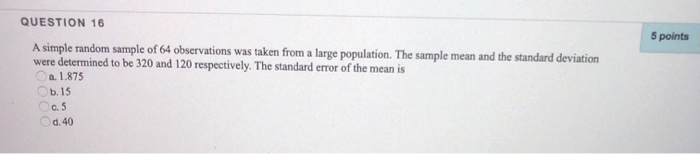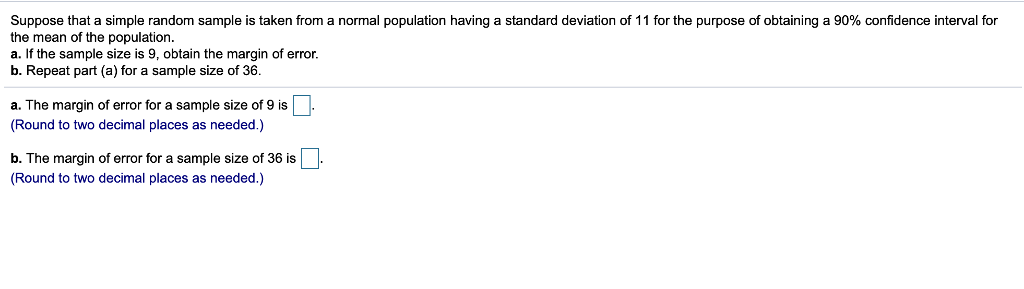Question

A simple random sample of 31 observations was taken from a large population. The sample mean equals 5. Five is a

 population parameter.
 standard error.
 point estimate.
 population mean.#### Earn Coins

Coins can be redeemed for fabulous gifts.

Similar Homework Help Questions
• ### A simple random sample of 64 observations was taken from a large population. The sample mean...

A simple random sample of 64 observations was taken from a large population. The sample mean and the standard deviation were determined to be 70 and 12 respectively. The standard error of the mean is . . . (hint: enter the answer with one decimal place)

• ### QUESTION 16 5 points A simple random sample of 64 observations was taken from a large...QUESTION 16 5 points A simple random sample of 64 observations was taken from a large population. The sample mean and the standard deviation were determined to be 320 and 120 respectively. The standard error of the mean is .1.875 .15 0.5 0.40

• ### Suppose that a simple random sample is taken from a normal population having a standard deviation...

Suppose that a simple random sample is taken from a normal population having a standard deviation of 15 for the purpose of obtaining a 95​% confidence interval for the mean of the population. a. If the sample size is 44​, obtain the margin of error. b. Repeat part​ (a) for a sample size of 25

• ### Suppose a simple random sample of 300 was taken from a large population. The sample had...Suppose a simple random sample of 300 was taken from a large population. The sample had 90% successes. For a 95% confidence level, find the following, rounded to the 4th decimal place. Use your T-Table to find the margin of error. a) How many successes were there? b) - c) d) Margin of Error = Points possible: 4 This is attempt 1 of 3. Message instructor about this question Submit

• ### Random samples of size 36 each are taken from a large population whose mean is 12...continues

Random samples of size 36 each are taken from a large population whose mean is 120 and standard deviation is 39. The mean and standard error of the samplingdistribution of sample mean, respectively, are:

• ### Suppose that a simple random sample is taken from a normal population having a standard deviation...

Suppose that a simple random sample is taken from a normal population having a standard deviation of 6 for the purpose of obtaining a 90​% confidence interval for the mean of the population. The margin of error for a sample size of 16 is ??? ​(Round to four decimal places as​ needed.) The margin of error for a sample size of 81 is ??? ​(Round to four decimal places as​ needed.)

• ### Suppose that a simple random sample is taken from a normal population having a standard deviation...

Suppose that a simple random sample is taken from a normal population having a standard deviation of 11 for the purpose of obtaining a 95​% confidence interval for the mean of the population. a. If the sample size is 16​, obtain the margin of error. b. Repeat part​ (a) for a sample size of 81. a. The margin of error for a sample size of 16 is ??? ​(Round to two decimal places as​ needed.) b. The margin of error...

• ### A sample of 49 observations is taken from a normal population with a standard deviation of 10. The sample mean is 55.

A sample of 49 observations is taken from a normal population with a standard deviation of 10. The sample mean is 55. Determine the 99% confidence interval for the population mean. (Round your answers to 2 decimal places.) Confidence interval for the population mean is _______ and _______ .A research firm conducted a survey to determine the mean amount Americans spend on coffee during a week. They found the distribution of weekly spending followed the normal distribution with a population standard deviation...

• ### Suppose that a simple random sample is taken from a normal population having a standard deviation...Suppose that a simple random sample is taken from a normal population having a standard deviation of 11 for the purpose of obtaining a 90% confidence interval for the mean of the population a. If the sample size is 9, obtain the margin of error. b. Repeat part (a) for a sample size of 36 a. The margin of error for a sample size of 9 is (Round to two decimal places as needed.) b. The margin of error for...

• ### A population has a mean of 300 and a standard deviation of 24. A simple random sample of 144...

A population has a mean of 300 and a standard deviation of 24. A simple random sample of 144 observations will be taken. What is the probability that the sample meanwill be between 297 and 303?